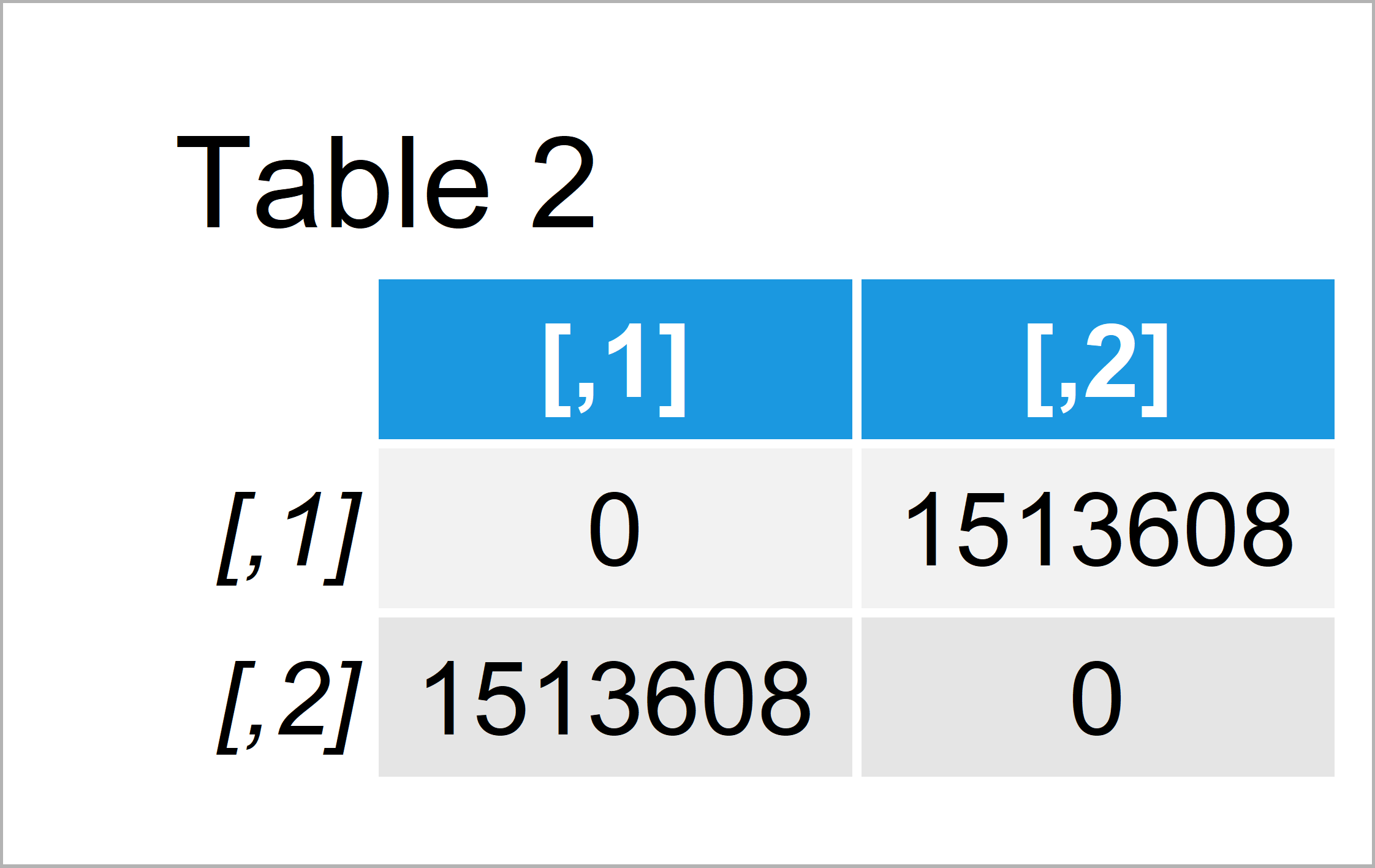# Geospatial Distance Between Two Points in R (Example)

In this article, I’ll illustrate how to calculate different types of geographical distance metrics in the R programming language.

The page looks as follows:

Here’s the step-by-step process.

## Constructing Example Data

The first step is to create some example data:

```my_points <- matrix(c(77.65465, 91.54323,    # Create longitude/latitude matrix
21.35444, 17.65465),
nrow = 2)
colnames(my_points) <- c("longitude", "latitude")
rownames(my_points) <- c("pt_1", "pt_2")
my_points                                    # Print longitude/latitude matrix```Table 1 shows the structure of our example data: It is composed of two geographical latitude and longitude points.

In this tutorial, I’ll show different ways on how to measure the geospatial distance between these two points in R.

Let’s do this!

## Example 1: Calculate Geospatial Distance Using geosphere Package

This example explains how to compute different measures of distance in the R programming language.

All distance metrics that I’ll explain in this tutorial are provided by the geosphere add-on package.

We first have to install and load the geosphere package to RStudio, if we want to use the corresponding functions:

```install.packages("geosphere")                # Install geosphere package

Now, we can use the different distance functions provided by the geosphere package. Besides others, we can calculate the Haversine distance

```distHaversine(my_points)                     # Calculate Haversine distance
#  1513707```
```distCosine(my_points)                        # Calculate Law of Cosines distance
#  1513707```

…the Meeus distance

```distMeeus(my_points)                         # Calculate Meeus distance
#  1513607```

…the distance along a rhumb line

```distRhumb(my_points)                         # Calculate rhumb distance
#  1514123```
```distVincentyEllipsoid(my_points)             # Calculate Vincenty ellipsoid distance
#  1513608```

…and the Vincenty sphere distance:

```distVincentySphere(my_points)                # Calculate Vincenty sphere distance
#  1513707```

Not all the previously used distance metrics return the same result. However, the differences between the metrics are not very large.

## Example 2: Calculate Geospatial Distance Matrix Using distm() Function

The geosphere package also provides the possibility to create a distance matrix. This is especially useful when you are dealing with many longitude and latitude points.

For this, we can use the distm function of the geosphere package.

In this example, I’ll explain how to calculate the distance on an ellipsoid by specifying the fun argument to be equal to distGeo. However, we may use any other function that I have explained in this tutorial as distance metric.

Let’s apply the distm function in R:

```dist_mat <- distm(my_points, fun = distGeo)  # Apply distm function
dist_mat                                     # Print distance matrix```After running the previous syntax the distance matrix shown in Table 2 has been created.

## Video & Further Resources

Would you like to know more about geospatial distances? Then I can recommend watching the following video of my YouTube channel. In the video, I’m explaining the content of this article:

Furthermore, you may have a look at the other articles about measuring geographical distances in R that I have published on my homepage:

Summary: This tutorial has illustrated how to compute geographical distance metrics in R programming. In case you have any additional questions, don’t hesitate to let me know in the comments section.

Subscribe to the Statistics Globe Newsletter

•February 11, 2021 10:57 pm

small error in syntax
distm(my_points, fun = distGeo) # Apply distm function
dist_mat # Print distance matrix

should be
dist_mat<-distm(my_points, fun = distGeo) # Apply distm function
dist_mat # Print distance matrix

•February 12, 2021 6:32 am

Hey Scott,

Thanks a lot for finding this error! 🙂 I have just updated the code.

Regards,

Joachim

•February 11, 2021 11:01 pm

is there any way to see the coding for the function distHaversine? My information about the Haversine formula is that it is pretty simple. However, I get a discrepancy between the result printed here and what I calculate with the Haversine formula. My result is 1544021 not 1513707 (I assume the output is meters).

•February 12, 2021 6:40 am

Hi again 🙂

Generally speaking: In RStudio, you can get the source code of every function by marking the function and pressing the F2 key (more information).

The distHaversine has the following source code:

```function (p1, p2, r = 6378137)
{
if (missing(p2)) {
p2 <- p1[-1, , drop = FALSE]
p1 <- p1[-nrow(p1), , drop = FALSE]
}
else {
}
p = cbind(p1[, 1], p1[, 2], p2[, 1], p2[, 2], as.vector(r))
dLat <- p[, 4] - p[, 2]
dLon <- p[, 3] - p[, 1]
a <- sin(dLat/2) * sin(dLat/2) + cos(p[, 2]) * cos(p[, 4]) *
sin(dLon/2) * sin(dLon/2)
a <- pmin(a, 1)
dist <- 2 * atan2(sqrt(a), sqrt(1 - a)) * p[, 5]
return(as.vector(dist))
}```

Regards,

Joachim

•Sapho
January 25, 2022 2:30 pm

Hello Joachim

Thanks for your post. I have a dataset with 60 000 points that depict a river course (pixel extracts from satellite image). The pixels are ordered ascendingly from source to outlet with an ID and each pixel has latitude and longitude. I need to know the distance in meters between two consecutive points. I fail to use distm() since it would result in a hugh matrix that exceeds the computer’s memory. How can I deal with this ? Many thanks.

•January 25, 2022 5:34 pm

Hey Sapho,

Thanks for the interesting comment!

Maybe you could create lagged variables for your latitude and longitude, and then you could use one of the apply functions to calculate the distm for each row?

Regards,
Joachim

•